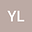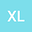Equivalence between invariant measures and statistical solutions for the 2D non-autonomous magneto-micropolar fluid equations
••Xiaojun Li
Hohai University
Author Profile## Abstract

In this article, we aim to investigate the regularity of statistical solution for the 2D non-autonomous magneto-micropolar fluid equations as well as the relationship between invariant measures and statistical solutions. Firstly, to get the regularity of the statistical solution, we prove the existence and regularity of the pullback attractor for the equations. Then we prove the statistical solution possesses some regularity properties by using regularity of the pullback attractor. Finally, we prove the statistical solution is actual an invariant measure for the equations.
29 May 2021Submitted to Mathematical Methods in the Applied Sciences
30 May 2021Submission Checks Completed
30 May 2021Assigned to Editor
08 Jun 2021Reviewer(s) Assigned
16 Sep 2021Review(s) Completed, Editorial Evaluation Pending
16 Sep 2021Editorial Decision: Revise Minor